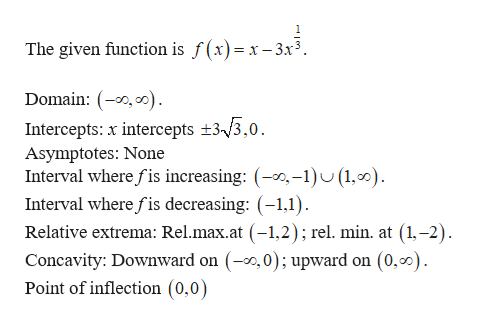# Use the information summarized below to sketch the graph of f. f(x) = x - 3 x^{1/3} Domain: (-∞, ∞)Intercepts: x-intercepts: ±3√3, 0Asymptotes: NoneIntervals where f is increasing: (-∞, -1) ∪ (1, ∞)Intervals where f is decreasing: (-1, 1)Relative extrema: Rel. max. at (-1, 2); rel. min. at (1, -2)Concavity: Downward on (-∞, 0); upward on (0, ∞)Point of inflection: (0, 0)

Question

Use the information summarized below to sketch the graph of f.
f(x) = x - 3 x^{1/3}

Domain: (-∞, ∞)
Intercepts: x-intercepts: ±3√3, 0
Asymptotes: None
Intervals where f is increasing: (-∞, -1) ∪ (1, ∞)
Intervals where f is decreasing: (-1, 1)
Relative extrema: Rel. max. at (-1, 2); rel. min. at (1, -2)
Concavity: Downward on (-∞, 0); upward on (0, ∞)
Point of inflection: (0, 0)

check_circle

Step 1

Given informa...help_outlineImage TranscriptioncloseThe given function is f(x)= x-3x3 Domain: (, Intercepts: x intercepts 3/3,0 Asymptotes: None Interval where fis increasing: -1)U (1,0) Interval where fis decreasing: (-1,1) Relative extrema: Rel.max.at (-1,2) ; rel. min. at (1,-2) Concavity: Downward on (,0); upward on (0,00) Point of inflection (0,0) fullscreen

### Want to see the full answer?

See Solution

#### Want to see this answer and more?

Solutions are written by subject experts who are available 24/7. Questions are typically answered within 1 hour.*

See Solution
*Response times may vary by subject and question.
Tagged in

### Other